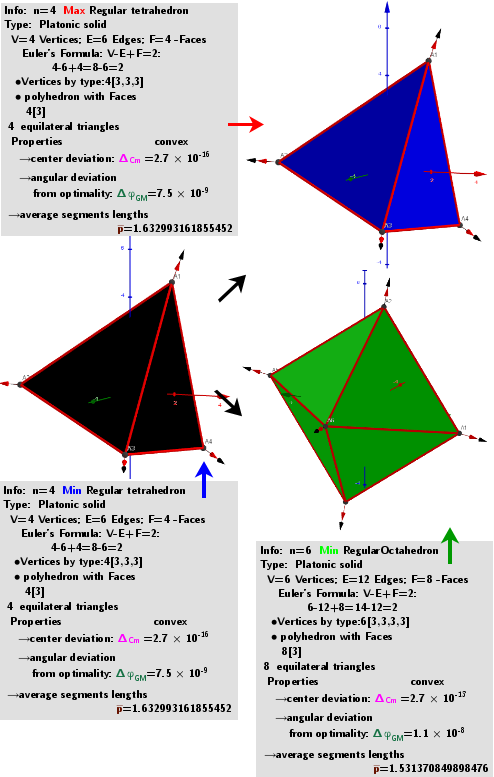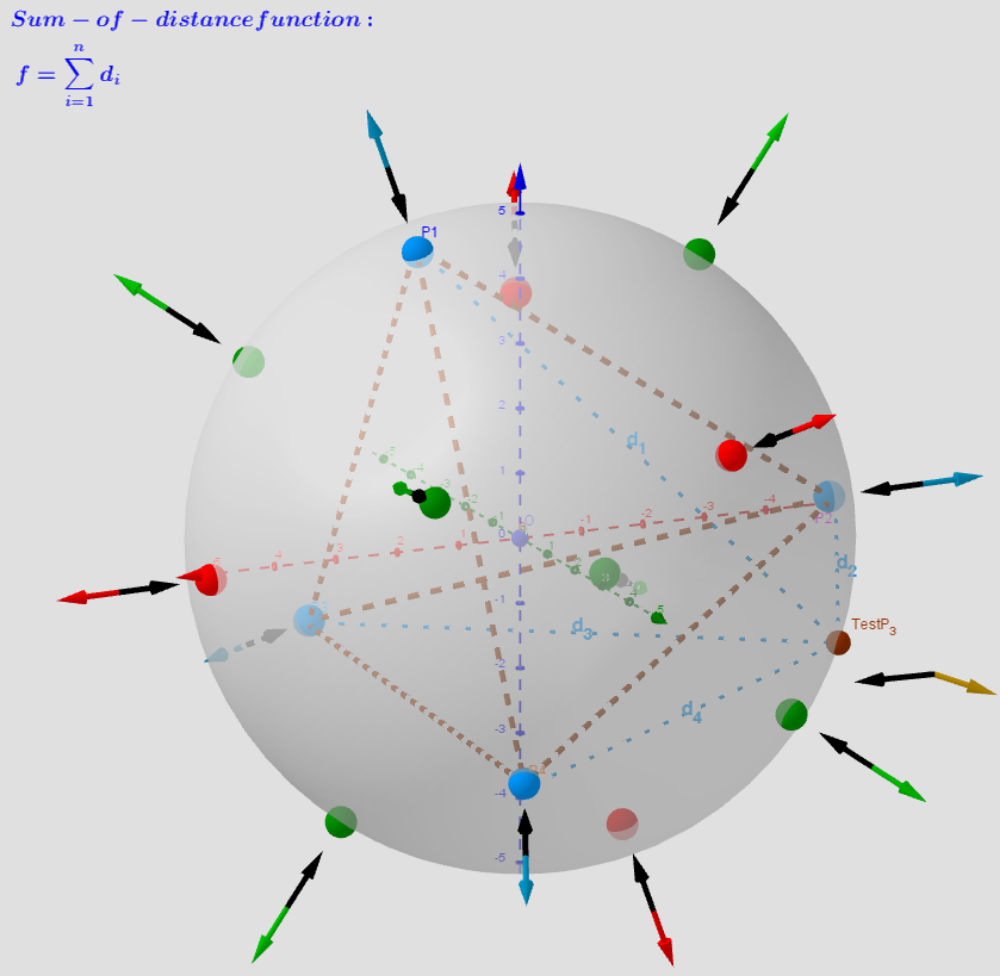# n=4 Tetrahedron. Images: A critical points scheme for Generating uniformly distributed points on a sphere.

The applet illustrates the case where 4 vertices of a regular tetrahedron "induce" the vertices of two other polyhedra: 4 ●Regular Tetrahedron4 ●Regular Tetrahedron6 ●Regular Octahedron. Description are in https://www.geogebra.org/m/y8dnkeuu and https://www.geogebra.org/m/rkpxwceh.A system of points on a sphere S of radius R “induces” on the sphere S0 of radius R0 three different sets of points, which are geometric medians (GM) -local maxima, minima and saddle points sum of distance function f(x). The angular coordinates of the spherical distribution of a system of points - local minima coincide with the original system of points.Distribution of points Pi, test Point, Max/min/saddle -Critical points on a sphere. Vectors ∇f and ∇g at these points.